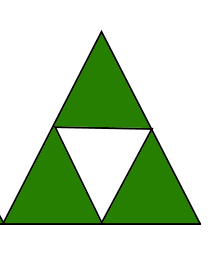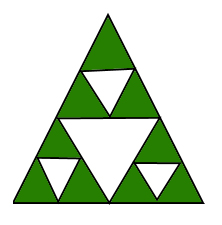# Number of triangles after N moves

Find the number of triangles in Nth step,
Rules: Draw an equilateral triangle at the start. In the i-th move, take the uncolored triangles, divides each of them in 4 parts of equal areas and color the central part. Keep a count of triangles till the Nth step.

Examples:

```Input : 1
Output : 5
Explanation: In 1st move we getInput : 2
Output : 17
Explanation: In 2nd move we get```

## Recommended: Please try your approach on {IDE} first, before moving on to the solution.

Naive approach:
The number of triangles in the nth figure are 3 times the number of triangles in the (n-1)th figure+2. We can see by observation the nth figure is made by placing 3 triangles similar to that in (n-1) figure and an inverted triangle. We also take into account the bigger triangle that has been formed. Hence the number of triangles in the nth figure becomes (number of triangles in the (n-1)th figure)*3 + 2.

## C++

 `// cpp program to calculate the number of equilateral ` `// triangles ` `#include ` `using` `namespace` `std; ` `// function to calculate number of triangles in Nth step ` `int` `numberOfTriangles(``int` `n) ` `{ ` `    ``int` `answer[n + 1] = { 0 }; ` `    ``answer = 1; ` `    ``for` `(``int` `i = 1; i <= n; i++)  ` `        ``answer[i] = answer[i - 1] * 3 + 2; ` `     `  `    ``return` `answer[n]; ` `} ` ` `  `// driver program  ` `int` `main() ` `{ ` `    ``int` `n = 2; ` `    ``cout << numberOfTriangles(n); ` `    ``return` `0; ` `} `

## Java

 `// Java program to find middle of three  ` `// distinct numbers to calculate the  ` `// number of equilateral triangles ` `import` `java.util.*; ` ` `  `class` `Triangle ` `{    ` `    ``// function to calculate number of  ` `    ``// traingles in Nth step ` `    ``public` `static` `int` `numberOfTriangles(``int` `n) ` `    ``{ ` `        ``int``[] answer = ``new` `int``[n+``1``]; ` `        ``answer[``0``] = ``1``; ` `         `  `        ``for` `(``int` `i = ``1``; i <= n; i++)  ` `            ``answer[i] = answer[i - ``1``] * ``3` `+ ``2``; ` `     `  `        ``return` `answer[n]; ` `    ``} ` `     `  `    ``// driver code ` `    ``public` `static` `void` `main(String[] args) ` `    ``{ ` `        ``int` `n = ``2``; ` `        ``System.out.println(numberOfTriangles(n)); ` `    ``} ` `} ` ` `  `// This code is contributed by rishabh_jain `

## Python3

 `# Python3 code to calculate the  ` `# number of equilateral triangles ` ` `  `# function to calculate number  ` `# of traingles in Nth step ` `def` `numberOfTriangles (n) : ` `    ``answer ``=` `[``None``] ``*` `(n ``+` `1``); ` `    ``answer[``0``] ``=` `1``; ` `    ``i ``=` `1` `    ``while` `i <``=` `n:  ` `        ``answer[i] ``=` `answer[i ``-` `1``] ``*` `3` `+` `2``; ` `        ``i ``=` `i ``+` `1` `     `  `    ``return` `answer[n]; ` ` `  `# Driver code ` `n ``=` `2` `print``(numberOfTriangles(n)) ` ` `  `# This code is contributed by "rishabh_jain". `

## C#

 `// C# program to find middle of three  ` `// distinct numbers to calculate the  ` `// number of equilateral triangles ` `using` `System; ` ` `  `class` `Triangle ` `{  ` `    ``// function to calculate number of  ` `    ``// traingles in Nth step ` `    ``public` `static` `int` `numberOfTriangles(``int` `n) ` `    ``{ ` `        ``int``[] answer = ``new` `int``[n+1]; ` `        ``answer = 1; ` `         `  `        ``for` `(``int` `i = 1; i <= n; i++)  ` `            ``answer[i] = answer[i - 1] * 3 + 2; ` `     `  `        ``return` `answer[n]; ` `    ``} ` `     `  `    ``// Driver code ` `    ``public` `static` `void` `Main() ` `    ``{ ` `        ``int` `n = 2; ` `        ``Console.WriteLine(numberOfTriangles(n)); ` `    ``} ` `} ` ` `  `// This code is contributed by vt_m `

## PHP

 ` `

Output:

```17
```

Time Complexity: O(n)

An efficient solution will be to derive a formula for Nth step:
If we follow the naive approach for every step, then we get for Nth step the number of triangles to be

(2*(3^n))-1.

## C++

 `// CPP program to calculate the number of  ` `// equilateral triangles ` `#include ` `using` `namespace` `std; ` ` `  `// function to calculate number of triangles  ` `// in Nth step ` `int` `numberOfTriangles(``int` `n) ` `{ ` `    ``int` `ans = 2 * (``pow``(3, n)) - 1; ` `    ``return` `ans; ` `} ` ` `  `// driver program  ` `int` `main() ` `{ ` `    ``int` `n = 2; ` `    ``cout << numberOfTriangles(n); ` `    ``return` `0; ` `} `

## Java

 `// Java program to find middle of three  ` `// distinct numbers to calculate the ` `// number of equilateral triangles ` `import` `java.util.*; ` `import` `static` `java.lang.Math.pow; ` ` `  `class` `Triangle ` `{    ` `    ``// function to calculate number  ` `    ``// of triangles in Nth step ` `    ``public` `static` `double` `numberOfTriangles(``int` `n) ` `    ``{ ` `        ``double` `ans = ``2` `* (pow(``3``, n)) - ``1``; ` `        ``return` `ans; ` `    ``} ` `     `  `    ``// driver code ` `    ``public` `static` `void` `main(String[] args) ` `    ``{ ` `        ``int` `n = ``2``; ` `        ``System.out.println(numberOfTriangles(n)); ` `    ``} ` `} ` ` `  `// This code is contributed by rishabh_jain `

## Python3

 `# Python3 code to calculate the  ` `# number of equilateral triangles ` ` `  `# function to calculate number ` `# of traingles in Nth step ` `def` `numberOfTriangles (n) : ` `    ``ans ``=` `2` `*` `(``pow``(``3``, n)) ``-` `1``; ` `    ``return` `ans; ` ` `  `# Driver code ` `n ``=` `2` `print` `(numberOfTriangles(n)) ` ` `  `# This code is contributed by "rishabh_jain". `

## C#

 `//C# program to find middle of three  ` `// distinct numbers to calculate the ` `// number of equilateral triangles ` `using` `System; ` ` `  `class` `Triangle ` `{  ` `    ``// function to calculate number  ` `    ``// of triangles in Nth step ` `    ``public` `static` `double` `numberOfTriangles(``int` `n) ` `    ``{ ` `        ``double` `ans = 2 * (Math.Pow(3, n)) - 1; ` `        ``return` `ans; ` `    ``} ` `     `  `    ``// Driver code ` `    ``public` `static` `void` `Main() ` `    ``{ ` `        ``int` `n = 2; ` `        ``Console.WriteLine(numberOfTriangles(n)); ` `    ``} ` `} ` ` `  `// This code is contributed by vt_m `

## PHP

 ` `

Output:

```17
```

Time Complexity: O(log n), log n is needed to compute 3^n.

Attention reader! Don’t stop learning now. Get hold of all the important DSA concepts with the DSA Self Paced Course at a student-friendly price and become industry ready.

My Personal Notes arrow_drop_upCheck out this Author's contributed articles.

If you like GeeksforGeeks and would like to contribute, you can also write an article using contribute.geeksforgeeks.org or mail your article to contribute@geeksforgeeks.org. See your article appearing on the GeeksforGeeks main page and help other Geeks.

Please Improve this article if you find anything incorrect by clicking on the "Improve Article" button below.

Improved By : vt_m, Akanksha_Rai